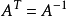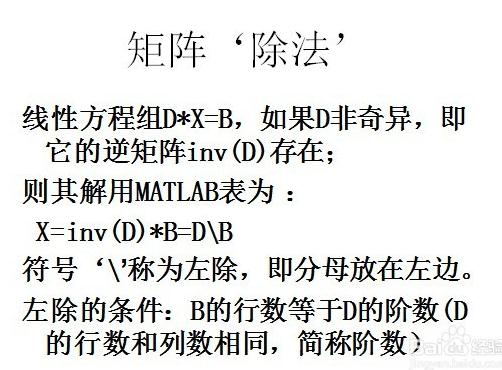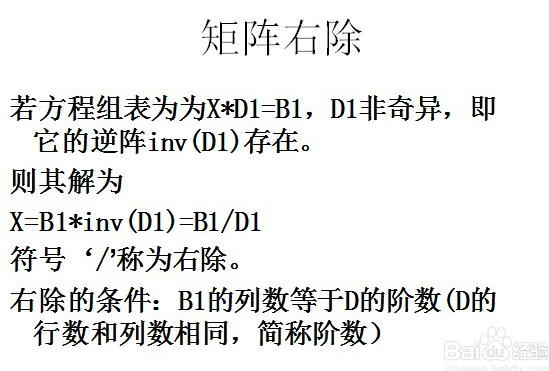• 矩阵的初等变换与初等矩阵的关系, 概括来说就是: 左行右列. 也就是说左乘初等矩阵进行的是行初等变换;而右乘初等矩阵进行的是列初等变换. 假设 Am×n=(a11a12...a1n............ai1ai2...ain............aj1aj2......
矩阵的初等变换与初等矩阵的关系, 概括来说就是: 左行右列. 也就是说左乘初等矩阵进行的是行初等变换;而右乘初等矩阵进行的是列初等变换.
假设
$A_{m\times n}=\begin{pmatrix} a_{11} & a_{12} & ... & a_{1n} \\ ... & ... & ... & ... \\ a_{i1} & a_{i2} & ... & a_{in} \\ ... & ... & ... & ... \\ a_{j1} & a_{j2} & ... & a_{jn} \\ ... & ... & ... & ... \\ a_{m1} & a_{m2} & ... & a_{mn} \\ \end{pmatrix}$
那么具体有如下几种情况:$AE(i,j(c))=\begin{pmatrix} a_{11}& ... & a_{1i} & ... & a_{1j}+ca_{1i} & ... & a_{1n} \\ a_{21}& ... & a_{2i} & ... & a_{2j}+ca_{2i} & ... & a_{2n} \\ ... & ... & ... & ... &... & ... & ... \\ a_{m1}& ... & a_{mi} & ... & a_{mj}+ca_{mi} & ... & a_{mn} \\ \end{pmatrix}$


展开全文线性代数
• 第三章，矩阵，06-初等变换与初等矩阵标准形初等行变换初等列变换初等变换行阶梯形矩阵行最简形标准形初等矩阵定义性质可逆等价矩阵相关定义行等价列等价等价性质定理1定理2推论 玩转线性代数(18)初等变换与初等矩阵...
第三章，矩阵，06-初等变换与初等矩阵标准形初等行变换初等列变换初等变换行阶梯形矩阵行最简形标准形初等矩阵定义性质可逆等价矩阵相关定义行等价列等价等价性质定理1定理2推论
玩转线性代数(18)初等变换与初等矩阵
的笔记
标准形
初等行变换
对矩阵的行进行对换、数乘(常数非零,下同)和倍加三种变换。
初等列变换
对矩阵的列进行对换、数乘(常数非零,下同)和倍加三种变换。
初等变换
初等行变换与初等列变换统称初等变换。
行阶梯形矩阵
初等行变换，至如下形式:
(1) 如果有零行，则位于矩阵的下方;
(2) 各非零行的首非零元的列标随着行标的增大而严格增大.
行最简形
首非零元为1和首非零元所在列其它元素为0的行阶梯形矩阵
标准形
标准形是对矩阵进行初等变换(一般在行最简形的基础上再进行初等列变换)将A化成如下形式：
$F=\begin{pmatrix} E_r & 0 \\ 0 & 0 \end{pmatrix}$,$\textbf{0}$为零矩阵，$r \leq m, r \leq n.$
初等矩阵
对矩阵进行一次初等变换相当于左乘了一个将单位矩阵进行了同样变换的矩阵。
定义
由单位矩阵E经过一次初等变换得到的矩阵称为初等矩阵。
初等矩阵共有三种类型：

互换两行或两列
互换E中第i,j两行/列的初等矩阵用$E_n(i,j)$表示
数乘
以数$k(\neq 0)$乘E的第i行/列($r_i×k$),用$E_n(i(k))$表示。
倍加
以k乘E的第j行加到第i行上($r_i+kr_j$),与以k乘E的第i列加到第j行上，
用$E_n(ij(k))$表示。
参见原文

性质
设A是一个m×n矩阵，
对A施行一次初等行变换，相当于在A的左边乘以相应的n阶初等矩阵。
对A施行一次初等列变换，相当于在A的右边乘以相应的n阶初等矩阵。
可逆
初等矩阵的逆变换就是对自身再做一次同类型的初等变换得到的矩阵。
$|E(i,j)|=-1;|E(i(j))|=k(k\neq 0);|E(i,j(k))|=1$，所以初等矩阵均可逆，由于
$E(i,j)E(i,j)=E, \\ E(i(k))E(i(\frac{1}{k}))=E,\\ E(ij(-k))E(ij(k))=E(ij(k))E(ij(-k))=E$
有：
$E(i,j)^{-1}=E(i,j), \\ E(i(k))^{-1}=E(i(\frac{1}{k})),\\ E(ij(k))^{-1}=E(ij(-k))$
等价矩阵
相关定义
行等价
矩阵A经有限次初等行变换化为B，则A与B行等价，记$A^r \sim B$
列等价
矩阵A经有限次初等列变换化为B，则A与B列等价，记$A^c \sim B$
等价
矩阵A经有限次初等变换化为B，则A与B等价，记$A \sim B$
性质
反身性：$A \sim A$;
对称性：若$A\sim B$,则$B \sim A$;
传递性：若$A\sim B,B \sim C$,则$A \sim C$
形式最简单的等价矩阵是标准形
定理1
设A与B为$m×n$矩阵，则

$A^r \sim B \Leftrightarrow p_l\cdots p_1 A=B \Leftrightarrow$ 存在m阶可逆矩阵P，使$PA=B$.
$A^c \sim B \Leftrightarrow$ 存在n阶可逆矩阵Q，使$AQ=B$.
$A \sim B \Leftrightarrow$ 分别存在m、n阶可逆矩阵P、Q，使$PAQ=B$.

定理2
设A为可逆矩阵，则存在有限个初等矩阵$P_1，P_2 ，\cdots ，P_l$，使$A=P_1P_2 \cdots P_l$
证
对可逆矩阵A进行初等变换，化为最简形F，
$p_1p_2...p_sAq_1q_2...q_t=F=\begin{pmatrix} E_r & 0 \\ 0 & 0 \end{pmatrix}_n$，故
$A=p_z^{-1}p_{z-1}^{-1}...p_1^{-1}\begin{pmatrix} E_r & 0 \\ 0 & 0 \end{pmatrix}_n q_t^{-1}q_{t-1}^{-1}...q_1^{-1}$
因为A可逆，所以F主对角线应没有零元，所以F=E。
所以
$A=p_z^{-1}p_{z-1}^{-1}...p_1^{-1} q_t^{-1}q_{t-1}^{-1}...q_1^{-1}$
例见原文
推论
方阵A可逆的充要条件是$A^c \sim E$或$A^r \sim E$
证明思路：
A可逆，则$A=P_1P_2 \cdots P_l$,所以A与E行等价或列等价；A与E行等价，则有$A=P_1P_2 \cdots P_lE$,可逆。列等价也一样。


展开全文• 初等矩阵 之前在解一个线性系统所创建的增广矩阵问题时，采用的主要是针对某一元素去执行操作 ,在矩阵的内部进行元素矩阵的操作==> 回忆：矩阵可以表示变换 上述的基本操作 能不能通过矩阵 来表示呢 ？ ==> 即...
初等矩阵
之前在解一个线性系统所创建的增广矩阵问题时，采用的主要是针对某一元素去执行操作 ,在矩阵的内部进行元素矩阵的操作==>回忆：矩阵可以表示变换
上述的基本操作 能不能通过矩阵 来表示呢 ？
==> 即：找到一个矩阵E 满足 E * A = A’
举例 之前学习的单位矩阵 ==>其实这个单位矩阵也可以看作是一个变换矩阵，只不过所作的变换是 没有变换 而已。
把 单位矩阵中的 元素 做出改变 >找到一个变换矩阵 ：让矩阵的一行 加（减）另一行
即满足>对应的变换矩阵 >找到一个变换矩阵 ：让矩阵的一行 减 第三行
即满足>对应的变换矩阵 >找到一个变换矩阵 ：让矩阵的一行 加（减） 另一行的若干倍
即满足>对应的变换矩阵 >找到一个变换矩阵 ：让矩阵的两行进行交换
即满足>对应的变换矩阵 ==>
改变两行，因为在结果矩阵中 需要改变两行。综上，矩阵的三个基本操作，都可以使用一个变换矩阵完成。矩阵的初等变换 ==>对应 矩阵的初等变换 的变换矩阵 ==> 初等矩阵 ==>初等矩阵：对单位矩阵进行依次初等变换得到的结果矩阵，记作E。
回忆使用 高斯-约旦消元法把矩阵化为行最简形式的过程。
==>
寻找一系列的初等矩阵E，使得：
Ep * … * E3 * E2 * E1 * A = rref（A）


展开全文线性代数
• 在做矩阵的逆运算（也就是出除法运算）时，分母的左右取决余原乘式左右； 右除式A/B，相当于A*inv(B)即A右乘B的逆矩阵； 左除式A\B，相当于inv（A）*B即A的逆矩阵左乘B ...


目录

正交矩阵;

实对称矩阵;

为什么实对称矩阵一定可以对角化;

AB=0 r(A)+r(B)<=n 证明;

初等矩阵;

初等矩阵的逆矩阵;

矩阵的左除右除;

正交矩阵;

如果：AAT=E（E为单位矩阵，AT表示“矩阵A的转置矩阵”。）或ATA=E，则n阶实矩阵A称为正交矩阵，若A为正交阵，则:

1)逆也是正交阵 对于一个正交矩阵来说，它的逆矩阵同样也是正交矩阵。

2)积也是正交阵 如果两个矩阵均为正交矩阵，那么它们的乘积也是正交矩阵。

3)A的各行是单位向量且两两正交

4)A的各列是单位向量且两两正交

5)(Ax,Ay)=(x,y)x,y∈R

6)|A|=1或-1

7)8)正交矩阵通常用字母Q表示。

实对称矩阵

如果有n阶矩阵A，其矩阵的元素都为实数，且矩阵A的转置等于其本身（aij=aji），(i,j为元素的脚标），则称A为实对称矩阵。

主要性质：

1.实对称矩阵A的不同特征值对应的特征向量是正交的。
2.实对称矩阵A的特征值都是实数，特征向量都是实向量。
3.n阶实对称矩阵A必可相似对角化，且相似对角阵上的元素即为矩阵本身特征值。
4.若A具有k重特征值λ0　必有k个线性无关的特征向量，或者说秩r(λ0E-A)必为n-k，其中E为单位矩阵。
5.实对称矩阵A一定可正交相似对角化。
为什么实对称矩阵一定可以对角化

实对称阵的特征值都是实数，所以n阶阵在实数域中就有n个特征值（包括重dao数），并且实对版称阵的每个特征值权的重数和属于它的无关的特征向量的个数是一样的，从而n阶矩阵共有n个无关特征向量，所以可对角化。

判断方阵是否可相似对角化的条件：

(1)充要条件：An可相似对角化的充要条件是：An有n个线性无关的特征向量；
(2)充要条件的另一种形式：An可相似对角化的充要条件是：An的k重特征值满足n-r(λE-A)=k；
(3)充分条件：如果An的n个特征值两两不同，那么An一定可以相似对角化；
(4)充分条件：如果An是实对称矩阵，那么An一定可以相似对角化。
AB=0 r(A)+r(B)<=n 证明：

AB=0，则说明B的列向量都是AX=0的解

因此B的列向量是AX=0解集的属子集

则B列向量组的秩，不大于方程组AX=0的基础解系的个数，即n-r(A)

即r(B)<= n-r(A)
因此：r(A)+r(B)<=n

初等矩阵

初等矩阵是指由单位矩阵经过一次初等变换得到的矩阵。初等矩阵的模样可以写一个3阶或者4阶的单位矩阵。

初等矩阵都可逆

初等矩阵的逆矩阵其实是一个同类型的初等矩阵（可看作逆变换）。

例如：

交换矩阵中某两行（列）的位置；
用一个非零常数k乘以矩阵的某一行（列）；
将矩阵的某一行（列）乘以常数k后加到另一行（列）上去。
若某初等矩阵左乘矩阵A，则初等矩阵会将原先施加到单位矩阵E上的变换，按照同种形式施加到矩阵A之上。或者说，想对矩阵A做变换，但是 不是直接对矩阵A去做处理，而是通过一种间接方式去实现。

初等矩阵的逆矩阵

1、行交换（列交换）的初等矩阵，逆矩阵还是本身；

2、某一行（或列）乘以一个倍数回的初等矩阵答，逆矩阵，是这一行（或列）除以这个倍数的初等矩阵；

3、某一行（或列）乘以一个倍数，加到另一行（或列）的初等矩阵，逆矩阵，是这一行（或列）乘以这个倍数的相反数，加到另外那一行（或列）的初等矩阵。

初等矩阵的逆矩阵其实是一个同类型的初等矩阵（可看作逆变换）。例如，交换矩阵中某两行（列）的位置；用一个非零常数k乘以矩阵的某一行（列）；将矩阵的某一行（列）乘以常数k后加到另一行（列）上去。

矩阵的左除右除

在做矩阵的逆运算（也就是出除法运算）时，分母的左右取决余原乘式左右；

右除式A/B，相当于A*inv(B)即A右乘B的逆矩阵；

左除式A\B，相当于inv（A）*B即A的逆矩阵左乘B展开全文• 文章目录矩阵分块法常用的分块法1) 按行分块2) 按列分块3) 分块对角矩阵(又称准对角矩阵)分块矩阵的运算分块矩阵的初等变换分块初等矩阵的性质参考 矩阵分块法 定义1\large\color{magenta}{\boxed{\color{brown}{...线性代数 自然语言处理 深度学习 数据挖掘 机器学习
• 初等矩阵和矩阵可逆性矩阵的LU分解概念LU分解过程LU分解的意义代码实现 矩阵的LU分解 概念 矩阵的分解实际上就是将一个矩阵分解成为几个矩阵乘积的形式。不同类型的矩阵分解有不同的目的，如矩阵的LU分解的目的是...
• 将方程化为如下的形式是高斯消元法的目标： {R=?G=?B=?\begin{cases} R=?\\G=?\\B=? \end{cases}⎩⎪⎨⎪⎧​R=?G=?B=?​ 思路： 首先利用第一行消去第二行和第三行的第一个元素： 接着利用第二行消去第三行的第二...
• 这不仅为几何变换空间透射找到简洁的解析形式，同时赋予数值计算工具初等矩阵以射影几何意义．由此出发，可重新解释包括Householder方法在内的一些线性代数方程组的直接解法，提出实际上是并行算法的焦平面法．通过...
• 初等矩阵和矩阵可逆性求解矩阵的逆矩阵的逆运算实现矩阵的逆 之前的笔记中曾提及过矩阵的逆的概念，但是并没有具体说明矩阵的逆的求法。 求解矩阵的逆 矩阵的逆运算 矩阵中AB=BA=IAB=BA=IAB=BA=I，则称B是A的逆矩阵...
• 注：本文是对Matrix Analysis and Applied ...在矩阵代数中，类似地，一个一般的矩阵也可能可以被分解成几个初等矩阵(Elementary Matrices)的乘积。 Matrices of the form I−uvT\mathbf I − \mathbf {uv}^TI−uvT, w线性代数
• ## 矩阵的初等变换

千次阅读 2016-10-13 09:26:31
Ei(k)E_i(k)：单位矩阵的第i行或者第i列乘以k倍得到的矩阵。 EijE_{ij}：单位矩阵第i行和第j行交换或者第i列和第j列交换得到的矩阵。 Eij(k)E_{ij}(k)：单位矩阵的第j行乘以k倍加到第i行，即被操作的行在前；那么...
• 矩阵初等变换的应用@(线性代数)这篇文章中介绍了矩阵初等变换的用法。http://blog.csdn.net/u011240016/article/details/52803938?locationNum=1&fps=1没有强调的是，左乘是行变换，右乘是列变换。三种形式六种...
• 常量矩阵变换,用于方便计算各矩阵初等变换, 结果以分数形式给出, 但是不能处理含有变量的矩阵 常量矩阵变换,用于方便计算各矩阵初等变换, 结果以分数形式给出, 但是不能处理含有变量的矩阵
• python实现用初等行变换将矩阵化为最简行形式 代码如下： # -*- coding: utf-8 -*- """ Created on Fri Mar 15 15:05:53 2019 @author: MrZjjPolarBear """ import numpy as np def rsmat(arbmat): """ ...
• 化成最简矩阵分成如下步骤 从矩阵的第 i=0 行开始化简 1.寻找最长元素行：从第i行开始，寻找第一个开头行元素非0的行，利用行交换把该行换到矩阵的第i行。 2.对第i行进行化简：对第i行的所有元素同除该行的首个非...C++ 数据结构
• import numpy as np def rsmat(arbmat): """ Convert an arbitrary matrix to a simplest matrix """ arbmat = arbmat.astype(float) row_number, column_number = arbmat.shape if row_number == 1: ...
• m]*arbmat[-1, :] break return arbmat #测试一下 a = np.matrix([ [1, 1, 0, -2, -6], [4, -1, -1, -1, 1], [3, -1, -1, 0, 3]]) a1 = rsmat(a) print(a1) 举个例子 此外，线性代数还有一个小工具矩阵计算器python numpy
• 矩阵初等变换这个概念可能在很多人听来有些陌生，但其实我们早在初中的解多元方程组的时候就用过它。只不过在课本当中，这种方法叫做消元法。我们先来看一个课本里的例子： {2x1−x2−x3+x4=2,(1)x1+x2−2x3+x4=4,...线性代数 算法
• 矩阵变换是线性代数中矩阵的一种运算形式。 在 线性代数 中，矩阵初等变换 是指以下三种变换类型 ： (1) 交换矩阵的两行（对调i,j，两行记为ri，rj）； (2) 以一个非零数k乘矩阵的某一行所有元素（第i行...python
• 设一个n级矩阵的全部初等因子为已知，在全部初等因子中将同一个一次因式的方幂的那些初等因子按降幂排列，而且当这些初等因子的个数不足n时，就在后面补上适当个数的1，使得凑成n个，设所得排列为...
• 代码如下 为何输出结果什么也没有？ #include<stdio.h> #define row 4 #define col 4 void main(void) { int det[row][col]={{1,2,3,4},{2,4,6,8},{2,0,4,6},{1,1,1,1}};...int r,c,i,flag=0,tmp=0;...
• 证明定义3：λ−\lambda-λ−矩阵初等变换定义4:等价定理2引理 定义1：秩 和数字矩阵的定义差不多 A(λ)A(\lambda)A(λ)中有一个r阶子式不为0, 而所有r+1阶子式为0⇒A(λ)\Rightarrow A(\lambda)⇒A(λ)的秩就为r...
• 一般，使用初等行变换来判定一个矩阵是否可逆，和求某矩阵的逆矩阵。 二阶矩阵使用伴随矩阵法比较方便，高阶矩阵使用初等行变换。 一般来说，将一个矩阵化为标准阵遵循下面方法：先用第一行消掉下面所有行的第一项...
• ...矩阵初等行变换下的标准型 ...一、矩阵初等行变换下的标准型---行标准型 m×n矩阵A= a11 a12 ... a1n a21 a22 ... a2n ... ... ... ... am1 am2 ... amn 的行标准形式是满足一算法 C
• 这篇文章和大家聊聊矩阵初等变换和矩阵的秩。矩阵初等变换这个概念可能在很多人听来有些陌生，但其实我们早在初中的解多元方程组的时候就用过它。只不过在课本当中，这种方法叫做消元法。我们先来看一个课本里的...
• 第3章 矩阵初等变换与线性方程组 3.1矩阵初等变换 矩阵初等变换是一种十分重要的运算，它在解线性方程组的解、求逆阵及矩阵理论的探索中都起重要的作用。 消元法解线性方程组 为引进矩阵初等变换，先来...线性代数 线性方程组...